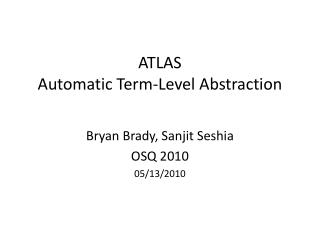DownloadDownload PresentationATLAS Automatic Term-Level Abstraction

# ATLAS Automatic Term-Level Abstraction

Télécharger la présentation## ATLAS Automatic Term-Level Abstraction

- - - - - - - - - - - - - - - - - - - - - - - - - - - E N D - - - - - - - - - - - - - - - - - - - - - - - - - - -
##### Presentation Transcript

1. ATLASAutomatic Term-Level Abstraction Bryan Brady, SanjitSeshia OSQ 2010 05/13/2010

2. HDLs are PLs • Verilog is a programming language • We want to prove certain properties about hardware (software) designs • Are two versions of a circuit (program) equivalent? • Does my circuit (program) satisfy property P?

3. Equivalence Checking ? = Circuit 1 Circuit 2 i0 i1 in i0 i1 in

4. Equivalence Checking (Can be hard!) ? * * = i0 i1 in i0 i1 in

5. Abstraction ? f f * * = i0 i1 in i0 i1 in

6. Abstraction Challenges • Hard to do manually even for small circuits/programs • Requires knowledge of circuit/program design • Can result in spurious counter-examples • How do we deal with this?

7. Automatic Abstraction • Combination of random simulation and static analysis • Identify candidate functional blocks for abstraction (modules/functions) using random simulation • For the functional blocks aren’t pruned in the random simulation phase, use static analysis to compute conditions under which it is precise to abstract

8. Computing Safe Abstractions

9. Promising Results

10. Question • Are there any software examples that might benefit from this technique?

11. Example Interpretation Condition Computation Initial State V16=F = out out 16 16 ALU ALU [19:16] [19:16] out_ok [15:0] [15:0] 16 V8=T 16 V15=T V7=T V6=F V14=F IMem IMem 4 4 20 20 PC PC V5=F V13=F = pc_ok +4 +4 V12=F 16 V4=F 16 = = 1 0 1 0 V3=T V11=T JMP JMP V2=T V10=T V1=F V9=T

12. Example Interpretation Condition Computation Initial State Update +4 Nodes V16=F = out out 16 16 ALU ALU [19:16] [19:16] out_ok [15:0] [15:0] 16 V8=T 16 V15=T V7=T V6=F V14=F IMem IMem 4 4 20 20 PC PC V5=T V5=F V13=F V13=T = pc_ok +4 +4 V12=F 16 V4=F 16 = = 1 0 1 0 V3=T V11=T JMP JMP V2=T V10=T V1=F V9=T

13. Example Interpretation Condition Computation Initial State Update +4 Nodes Update PC Latch Nodes V16=F = out out 16 16 ALU ALU [19:16] [19:16] out_ok [15:0] [15:0] 16 V8=T 16 V15=T V7=T V6=F V14=F IMem IMem 4 4 20 20 PC PC V5=T V13=T = pc_ok +4 +4 V12=T V12=F 16 V4=F V4=T 16 = = 1 0 1 0 V3=T V11=T JMP JMP V2=T V10=T V1=F V9=T

14. Example Interpretation Condition Computation Initial State Update +4 Nodes Update PC Latch Nodes Update ITE Nodes V16=F = out out 16 16 ALU ALU [19:16] [19:16] out_ok [15:0] [15:0] 16 V8=T 16 V15=T V7=T V6=F V14=F IMem IMem 4 4 20 20 PC PC V5=T V13=T = pc_ok +4 +4 V12=T 16 V4=T 16 = = 1 0 1 0 V3=T V11=T JMP JMP V2=T V10=T V1=JMP==instr[19:16] V1=F V9=T

15. Example Interpretation Condition Computation Initial State Update +4 Nodes Update PC Latch Nodes Update ITE Nodes Update out_ok Node V16=JMP==instr[19:16] V16=F = out out 16 16 ALU ALU [19:16] [19:16] out_ok [15:0] [15:0] 16 V8=T 16 V15=T V7=T V6=F V14=F IMem IMem 4 4 20 20 PC PC V5=T V13=T = pc_ok +4 +4 V12=T 16 V4=T 16 = = 1 0 1 0 V3=T V11=T JMP JMP V2=T V10=T V1=JMP==instr[19:16] V9=T